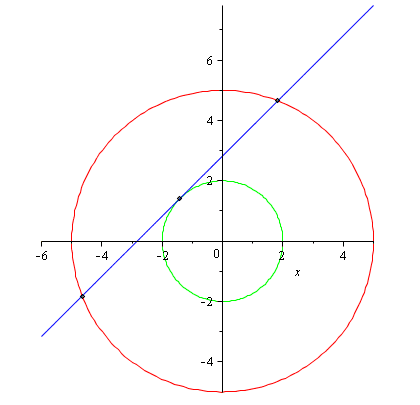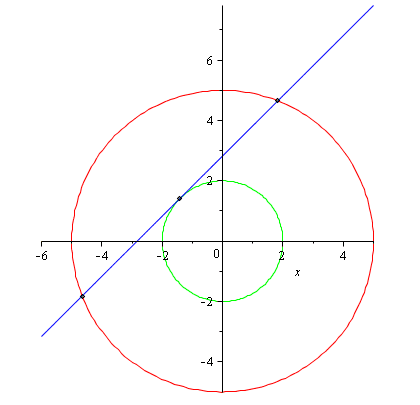# Finding intersection of line and circle.

## Homework Statement

I have a line obtained from using a slope of 1 and point (-sqrt(2),sqrt(2)):

y - sqrt(2) = 1 (x+sqrt(2))
y = 2*sqrt(2) + x

and a circle with radius 5 centered at origin.

My thought was to solve this parametricallyThe line is the tangent line (blue line). I am trying to find the insersections (points) where the blue line meets the red circle.

## The Attempt at a Solution

Given point (-sqrt(2),sqrt(2)) and knowing the vector <1,1>

X = -sqrt(2)+t
Y = sqrt(2)+t

X = 5 * cos(t)
Y = 5 * sin(t)

Subsituting:

-sqrt(2)+t = 5 * cos(t)
sqrt(2)+t = 5 * sin(t)

I then attempt to solve for t, but cannot find a solution.

LCKurtz
Homework Helper
Gold Member

## Homework Statement

I have a line obtained from using a slope of 1 and point (-sqrt(2),sqrt(2)):

y - sqrt(2) = 1 (x+sqrt(2))
y = 2*sqrt(2) + x

and a circle with radius 5 centered at origin.

My thought was to solve this parametricallyThe line is the tangent line (blue line). I am trying to find the insersections (points) where the blue line meets the red circle.

## The Attempt at a Solution

Given point (-sqrt(2),sqrt(2)) and knowing the vector <1,1>

X = -sqrt(2)+t
Y = sqrt(2)+t

X = 5 * cos(t)
Y = 5 * sin(t)

Subsituting:

-sqrt(2)+t = 5 * cos(t)
sqrt(2)+t = 5 * sin(t)

I then attempt to solve for t, but cannot find a solution.

Try squaring those last two equations and adding them.

LCKurtz
Homework Helper
Gold Member
-sqrt(2)+t = 5 * cos(t)
sqrt(2)+t = 5 * sin(t)

I then attempt to solve for t, but cannot find a solution.

Try squaring those last two equations and adding them.

What part about those "last two equations" don't you understand?

What part about those "last two equations" don't you understand?

How it relates to any mathematical formula or understanding, taking your reply literally :

(-sqrt(2)+t)^2 + (sqrt(2)+t)^2 = (5 * cos(t))^2 + (5 * sin(t))^2

Yielded nothing useful.

LCKurtz
Homework Helper
Gold Member
How it relates to any mathematical formula or understanding, taking your reply literally :

(-sqrt(2)+t)^2 + (sqrt(2)+t)^2 = (5 * cos(t))^2 + (5 * sin(t))^2

Yielded nothing useful.

So simplify it. Do some algebra.

So simplify it. Do some algebra.

http://www.wolframalpha.com/input/?...qrt(2)+t)^2+=+(5+*+cos(t))^2+++(5+*+sin(t))^2

Gives me t= ± sqrt(21/2)

Pluggin this into

X= 5*cos(t), gives ~ -4.97

Of which according to maple the approx points of X I am looking for are 1.826 and -4.654

-----------

If you would please explain to me the mathematical formula you were apply when squaring both and adding both equations

Edit:

Using

X = -sqrt(2) ± sqrt(21/2) does indeed yield the points of X I am looking for, however I am a bit confused why the parametic equation from the circle does not.

I am not looking for a cut and dry tell me the solution answer. I am more interested in actually -learning- how it was done. At this point I don't understand why you did what you did worked.

Last edited:
LCKurtz
Homework Helper
Gold Member
I am not looking for a cut and dry tell me the solution answer. I am more interested in actually -learning- how it was done. At this point I don't understand why you did what you did worked.

Good. Then let's see your work simplifying$$(-\sqrt{2}+t)^2 + (\sqrt{2}+t)^2 = (5 * \cos(t))^2 + (5 * \sin(t))^2$$Show your steps. When you simplify it you will see why it works and why I suggested squaring and adding.

I have to leave now, be back later this afternoon

Good. Then let's see your work simplifying$$(-\sqrt{2}+t)^2 + (\sqrt{2}+t)^2 = (5 * \cos(t))^2 + (5 * \sin(t))^2$$Show your steps. When you simplify it you will see why it works and why I suggested squaring and adding.

I have to leave now, be back later this afternoon

$\left( -\sqrt {2}+t \right) ^{2}+ \left( \sqrt {2}+t \right) ^{2}=25 \, \left( \cos \left( t \right) \right) ^{2}+25\, \left( \sin \left( t \right) \right) ^{2}$

$\left( -\sqrt {2}+t \right) ^{2}+ \left( \sqrt {2}+t \right) ^{2}=25( \, \left( \cos \left( t \right) \right) ^{2}+\left( \sin \left( t \right) \right) ^{2})$

$\left( -\sqrt {2}+t \right) ^{2}+ \left( \sqrt {2}+t \right) ^{2}=25(1)$

$\left( -\sqrt {2}+t \right) ^{2}+ \left( \sqrt {2}+t \right) ^{2}=25$

$(2+2\sqrt{2}t+t^{2})+(2-2\sqrt{2}t+t^{2}) = 25$

$4+2t^{2} = 25$

${t}^{2}=\frac{21}{2}$

${t}=±\sqrt{\frac{21}{2}}$

I understand basically in the most simplistic sense you did

x(t)^2+y(t)^2 = x1(t)^2+y1(t)^2

Which will should still give a t value that satisfies what I needed, you squared it for the trig identity cos^2 + sin^2 = 1 to cancel out the trig, which messed up my equation when trying to solve for it. It seems there was no known mathematical procedure or formula that unique or specific to this type of problem but rather intuition was used to make the problem solvable, which was not as obvious to me.

Still for this value of T you find using x(t)^2+y(t)^2 = x1(t)^2+y1(t)^2 it doesnt seem to provide

x(t) = x1(t) and y(t)=y1(t)

-------------

Am I correct in my understanding?

LCKurtz
Homework Helper
Gold Member
$\left( -\sqrt {2}+t \right) ^{2}+ \left( \sqrt {2}+t \right) ^{2}=25 \, \left( \cos \left( t \right) \right) ^{2}+25\, \left( \sin \left( t \right) \right) ^{2}$

$\left( -\sqrt {2}+t \right) ^{2}+ \left( \sqrt {2}+t \right) ^{2}=25( \, \left( \cos \left( t \right) \right) ^{2}+\left( \sin \left( t \right) \right) ^{2})$

$\left( -\sqrt {2}+t \right) ^{2}+ \left( \sqrt {2}+t \right) ^{2}=25(1)$

$\left( -\sqrt {2}+t \right) ^{2}+ \left( \sqrt {2}+t \right) ^{2}=25$

$(2+2\sqrt{2}t+t^{2})+(2-2\sqrt{2}t+t^{2}) = 25$

$4+2t^{2} = 25$

${t}^{2}=\frac{21}{2}$

${t}=±\sqrt{\frac{21}{2}}$

I understand basically in the most simplistic sense you did

x(t)^2+y(t)^2 = x1(t)^2+y1(t)^2

Which will should still give a t value that satisfies what I needed, you squared it for the trig identity cos^2 + sin^2 = 1 to cancel out the trig, which messed up my equation when trying to solve for it. It seems there was no known mathematical procedure or formula that unique or specific to this type of problem but rather intuition was used to make the problem solvable, which was not as obvious to me.

Still for this value of T you find using x(t)^2+y(t)^2 = x1(t)^2+y1(t)^2 it doesnt seem to provide

x(t) = x1(t) and y(t)=y1(t)

-------------

Am I correct in my understanding?

Yes. And the sine and cosine terms are why we squared to get rid of them. All that remains to do is to use your t values in your original equations:
##x = -\sqrt{2}+t##
##y = \sqrt{2}+t##
Put ##t = \sqrt{\frac {21} 2}## in to get one ##(x,y)## point and ##t = -\sqrt{\frac {21} 2}## in the other to get the other one.

You might notice that instead of messing with the parametric equation of the circle, you could have just substituted the x and y parametric equations into ##x^2+y^2= 25## eliminating the need for the trig functions.